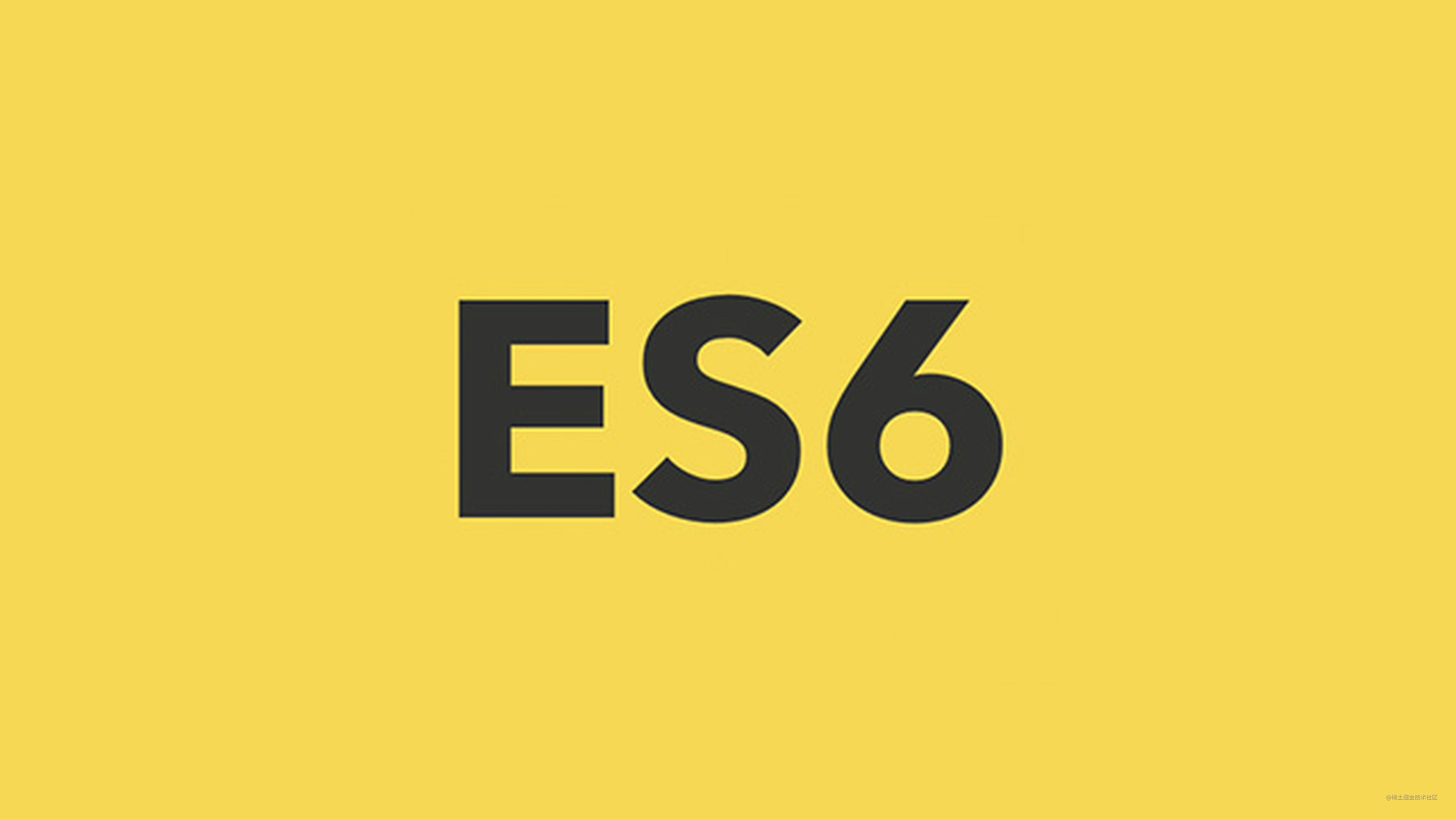# ES6 系列之模块加载方案1. AMD
2. CMD
3. CommonJS
4. ES6 模块

## require.js

``````* project/
* index.html
* vender/
* main.js
* require.js
* square.js
* multiply.js

`index.html` 的内容如下：

``````<!DOCTYPE html>
<html>
<title>require.js</title>
<body>
<h1>Content</h1>
<script data-main="vender/main" src="vender/require.js"></script>
</body>
</html>

`data-main="vender/main"` 表示主模块是 `vender` 下的 `main.js`

`main.js` 的配置如下：

``````// main.js
console.log(squareModule.square(3))
});

require 的第一个参数表示依赖的模块的路径，第二个参数表示此模块的内容。

``````// add.js
define(function() {
var add = function(x, y) {&emsp;
return x + y;
};

return {&emsp;&emsp;&emsp;&emsp;&emsp;&emsp;
};
});

`requirejs` 为全局添加了 `define` 函数，你只要按照这种约定的方式书写这个模块即可。

``````// multiply.js
define(function() {
console.log('加载了 multiply 模块')
var multiply = function(x, y) {&emsp;
return x * y;
};

return {&emsp;&emsp;&emsp;&emsp;&emsp;&emsp;
multiply: multiply
};
});

`square 模块`就要用到 `multiply 模块`，其实写法跟 main.js 添加依赖模块一样：

``````// square.js
define(['./multiply'], function(multiplyModule) {
console.log('加载了 square 模块')
return {&emsp;&emsp;&emsp;&emsp;&emsp;&emsp;
square: function(num) {
return multiplyModule.multiply(num, num)
}
};
});

require.js 会自动分析依赖关系，将需要加载的模块正确加载。

requirejs 项目 Demo 地址：github.com/mqyqingfeng…

``````加载了 add 模块

2
9

## AMD

`requirejs` 为全局添加了 `define` 函数，你只要按照这种约定的方式书写这个模块即可。

## sea.js

``````* project/
* index.html
* vender/
* main.js
* require.js
* square.js
* multiply.js

`index.html` 的内容如下：

``````<!DOCTYPE html>
<html>
<title>sea.js</title>
<body>
<h1>Content</h1>
<script src="vender/sea.js"></script>
<script>
// 在页面中加载主模块
seajs.use("./vender/main");
</script>
</body>

</html>

main.js 的内容如下：

``````// main.js
define(function(require, exports, module) {

var squareModule = require('./square');
console.log(squareModule.square(3))
});

``````// add.js
define(function(require, exports, module) {
var add = function(x, y) {&emsp;
return x + y;
};
module.exports = {&emsp;&emsp;&emsp;&emsp;&emsp;&emsp;
};
});

square.js 的内容如下：

``````define(function(require, exports, module) {
console.log('加载了 square 模块')
var multiplyModule = require('./multiply');
module.exports = {&emsp;&emsp;&emsp;&emsp;&emsp;&emsp;
square: function(num) {
return multiplyModule.multiply(num, num)
}
};

});

multiply.js 的内容如下：

``````define(function(require, exports, module) {
console.log('加载了 multiply 模块')
var multiply = function(x, y) {&emsp;
return x * y;
};
module.exports = {&emsp;&emsp;&emsp;&emsp;&emsp;&emsp;
multiply: multiply
};
});

seajs 项目 Demo 地址：github.com/mqyqingfeng…

``````加载了 add 模块
2

9

## AMD 与 CMD 的区别

1.CMD 推崇依赖就近，AMD 推崇依赖前置。看两个项目中的 main.js：

``````// require.js 例子中的 main.js
// 依赖必须一开始就写好
console.log(squareModule.square(3))
});

``````// sea.js 例子中的 main.js
define(function(require, exports, module) {

// 依赖可以就近书写
var squareModule = require('./square');
console.log(squareModule.square(3))
});

2.对于依赖的模块，AMD 是提前执行，CMD 是延迟执行。看两个项目中的打印顺序：

``````// require.js

2
9

``````// sea.js

2

9

AMD 是将需要使用的模块先加载完再执行代码，而 CMD 是在 require 的时候才去加载模块文件，加载完再接着执行。

## CommonJS

AMD 和 CMD 都是用于浏览器端的模块规范，而在服务器端比如 node，采用的则是 CommonJS 规范。

``````var add = function(x, y) {&emsp;
return x + y;
};

``````var add = require('./add.js');

``````// main.js

var square = require('./square.js');
console.log(square.square(3));

``````// add.js

var add = function(x, y) {&emsp;
return x + y;
};

``````// multiply.js
console.log('加载了 multiply 模块')

var multiply = function(x, y) {&emsp;
return x * y;
};

module.exports.multiply = multiply;

``````// square.js
console.log('加载了 square 模块')

var multiply = require('./multiply.js');

var square = function(num) {&emsp;
return multiply.multiply(num, num);
};

module.exports.square = square;

CommonJS 项目 Demo 地址：github.com/mqyqingfeng…

``````加载了 add 模块
2

9

## CommonJS 与 AMD

CommonJS 规范加载模块是同步的，也就是说，只有加载完成，才能执行后面的操作。

AMD规范则是非同步加载模块，允许指定回调函数。

## ES6

ECMAScript2015 规定了新的模块加载方案。

``````var firstName = 'Michael';
var lastName = 'Jackson';
var year = 1958;

export {firstName, lastName, year};

``````import {firstName, lastName, year} from './profile';

``````<!DOCTYPE html>
<html>
<title>ES6</title>
<body>
<h1>Content</h1>
<script src="vender/main.js" type="module"></script>
</body>
</html>

``````// main.js

import {square} from './square.js';
console.log(square(3));

``````// add.js

var add = function(x, y) {
return x + y;
};

``````// multiply.js
console.log('加载了 multiply 模块')

var multiply = function(x, y) {&emsp;
return x * y;
};

export {multiply}

``````// square.js
console.log('加载了 square 模块')

import {multiply} from './multiply.js';

var square = function(num) {&emsp;
return multiply(num, num);
};

export {square}

ES6-Module 项目 Demo 地址：github.com/mqyqingfeng…

``````cnpm install http-server -g

``````http-server

``````加载了 add 模块

2
9

## ES6 与 CommonJS

1. CommonJS 模块输出的是一个值的拷贝，ES6 模块输出的是值的引用。

2. CommonJS 模块是运行时加载，ES6 模块是编译时输出接口。

CommonJS 模块输出的是值的拷贝，也就是说，一旦输出一个值，模块内部的变化就影响不到这个值。

``````// 输出模块 counter.js
var counter = 3;
function incCounter() {
counter++;
}
module.exports = {
counter: counter,
incCounter: incCounter,
};

``````// 引入模块 main.js
var mod = require('./counter');

console.log(mod.counter);  // 3
mod.incCounter();
console.log(mod.counter); // 3

counter.js 模块加载以后，它的内部变化就影响不到输出的 mod.counter 了。这是因为 mod.counter 是一个原始类型的值，会被缓存。

``````// 输出模块 counter.js
var counter = {
value: 3
};

function incCounter() {
counter.value++;
}
module.exports = {
counter: counter,
incCounter: incCounter,
};

``````// 引入模块 main.js
var mod = require('./counter.js');

console.log(mod.counter.value); // 3
mod.incCounter();
console.log(mod.counter.value); // 4

value 是会发生改变的。不过也可以说这是 "值的拷贝"，只是对于引用类型而言，值指的其实是引用。

``````// counter.js
export let counter = 3;
export function incCounter() {
counter++;
}

// main.js
import { counter, incCounter } from './counter';
console.log(counter); // 3
incCounter();
console.log(counter); // 4

ES6 模块的运行机制与 CommonJS 不一样。JS 引擎对脚本静态分析的时候，遇到模块加载命令 import，就会生成一个只读引用。等到脚本真正执行时，再根据这个只读引用，到被加载的那个模块里面去取值。换句话说，ES6 的 import 有点像 Unix 系统的“符号连接”，原始值变了，import 加载的值也会跟着变。因此，ES6 模块是动态引用，并且不会缓存值，模块里面的变量绑定其所在的模块。

## Babel

``````// ES6
var firstName = 'Michael';
var lastName = 'Jackson';
var year = 1958;

export {firstName, lastName, year};

``````// Babel 编译后
'use strict';

Object.defineProperty(exports, "__esModule", {
value: true
});
var firstName = 'Michael';
var lastName = 'Jackson';
var year = 1958;

exports.firstName = firstName;
exports.lastName = lastName;
exports.year = year;

``````// ES6
import {firstName, lastName, year} from './profile';

``````// Babel 编译后
'use strict';

var _profile = require('./profile');

## webpack

Babel 将 ES6 模块转为 CommonJS 后， webpack 又是怎么做的打包的呢？它该如何将这些文件打包在一起，从而能保证正确的处理依赖，以及能在浏览器中运行呢？

``````console.log('加载了 square 模块')

var multiply = require('./multiply.js');

var square = function(num) {&emsp;
return multiply.multiply(num, num);
};

module.exports.square = square;

webpack 会将其包裹一层，注入这些变量：

``````function(module, exports, require) {
console.log('加载了 square 模块');

var multiply = require("./multiply");
module.exports = {
square: function(num) {
return multiply.multiply(num, num);
}
};
}

``````// 自执行函数
(function(modules) {

// 用于储存已经加载过的模块
var installedModules = {};

function require(moduleName) {

if (installedModules[moduleName]) {
return installedModules[moduleName].exports;
}

var module = installedModules[moduleName] = {
exports: {}
};

modules[moduleName](module, module.exports, require);

return module.exports;
}

// 加载主模块
return require("main");

})({
"main": function(module, exports, require) {

var squareModule = require("./square");
console.log(squareModule.square(3));

},
"./add": function(module, exports, require) {

module.exports = {
add: function(x, y) {
return x + y;
}
};
},
"./square": function(module, exports, require) {
console.log('加载了 square 模块');

var multiply = require("./multiply");
module.exports = {
square: function(num) {
return multiply.multiply(num, num);
}
};
},

"./multiply": function(module, exports, require) {
console.log('加载了 multiply 模块');

module.exports = {
multiply: function(x, y) {
return x * y;
}
};
}
})

``````加载了 add 模块
2

9

## ES6 系列

ES6 系列目录地址：github.com/mqyqingfeng…

ES6 系列预计写二十篇左右，旨在加深 ES6 部分知识点的理解，重点讲解块级作用域、标签模板、箭头函数、Symbol、Set、Map 以及 Promise 的模拟实现、模块加载方案、异步处理等内容。